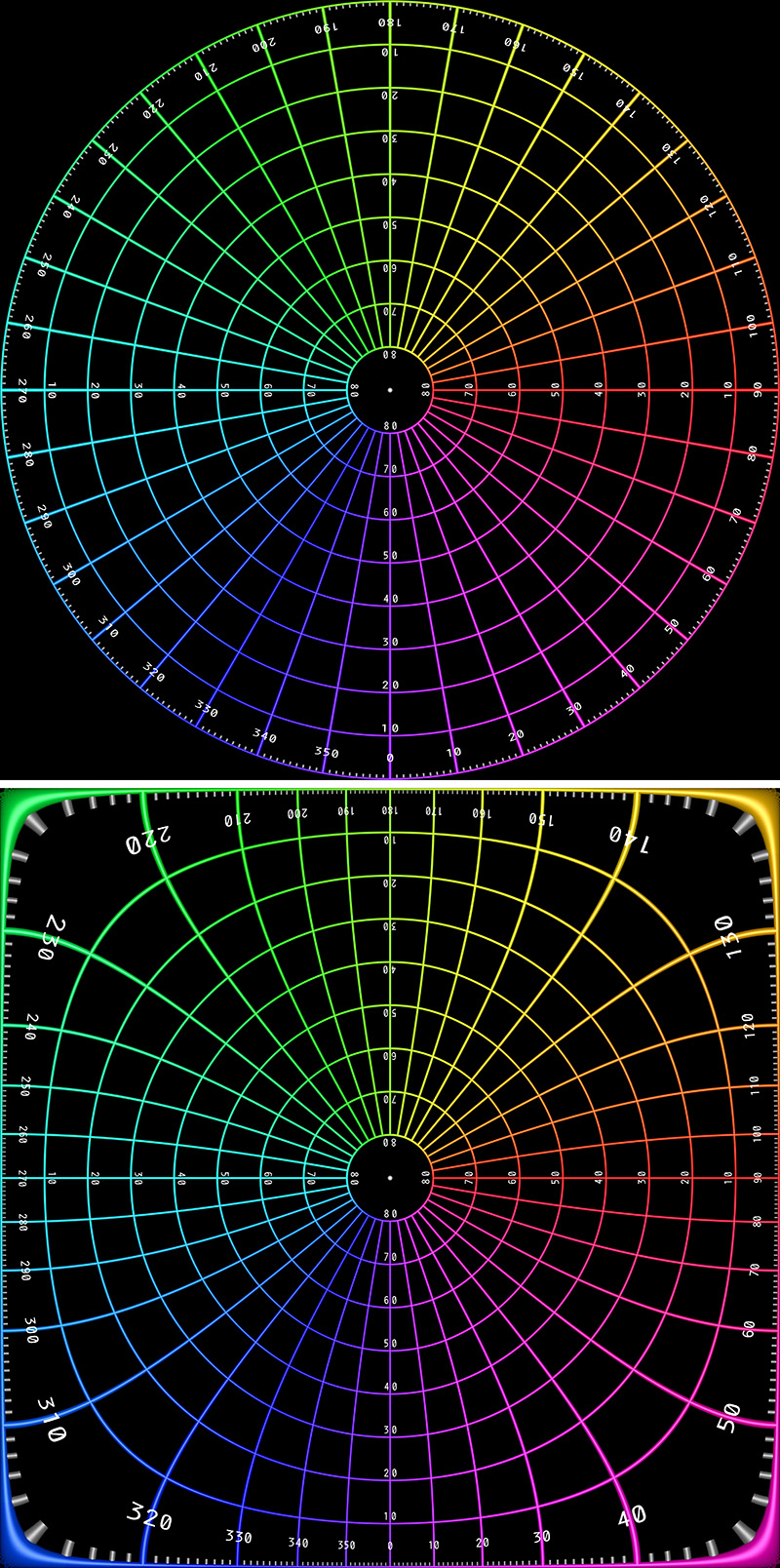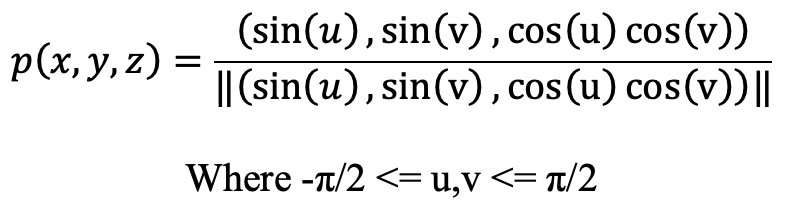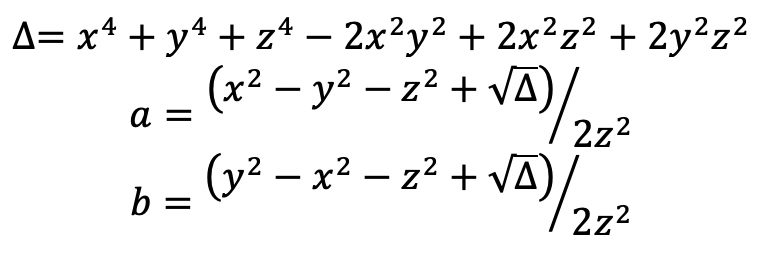# Square Fisheye

Written by Paul Bourke
Special tanks to Nan Ma for solving the inverse mapping equations
January 2020

The following introduces the concept of a 180 degree fisheye (although other angles are possible) that fills a square image, rather than being limited to an inscribed circle as per the usual fisheye projection. The method presented here is just one of a large number of ways a circle can be expanded to fill a square.

In the transformation here the field angle p(x,y,z) for every point (u,v) in a square image plane is given below. This mapping is conformal except for the 4 corner points of the square, that is, angles between features in the input image are preserved in the output image. The mapping is not equal area.A 180 degree fisheye is represented in the traditional way in the first image below, the equivalent square fisheye is shown in the second image. For reasons that are not at all obvious, the MandelBulb3D software offers this as one of the rendering options, specifically, the "common" camera with 180 field of view.This projection has been discussed in papers by Chamberlain Fong under the somewhat more complex formulation called Schwarz-Christoffel mapping, credited to Hermann Schwarz and Elwin Christoffel. Unfortunately it uses complex analysis and the formulation uses rather complicated complex valued incomplete Legendre elliptic integrals of the 1st kind as well as complex valued Jacobi elliptic functions.

The forward mapping can be expressed as a transformation from a 2D point on the image plane (u,v) to a unit vector p(x,y,z). Conversions between a circular fisheye image and square fisheye image is implemented as follows.Where p(x,y,z) is the field angle for a particular pixel (u,v). The inverse mapping, solved by Nan Ma, is given below. Specifically, given a unit vector (x,y,z) what is the corresponding (u,v).

Define the followingthenThe forward and reverse mappings have been implemented in a command line style utility that can convert to and from standard circular fisheyes and square fisheyes. Please note that in this case the solution assumes the mappings are between vectors p(x,y,z) encoded as fisheye projections on the image plane.

```Usage: square2fish [options] squareimage
Options
-w n       width and height of the fisheye image, default = 1024
-t n       fisheye FOV (degrees), default = 180
-a n       antialiasing level, default = 2
-o s       output file name, default: name derived from input filename
-d n       direction, 1 or -1, default: 1
-v         debug mode, default: off
```
References

• Analytical Methods for Squaring the Disc. Chamberlain Fong. Seoul ICM 2014.

• Elliptification of Rectangular Imagery. Chamberlain Fong. Joint Mathematics Meetings 2019, SIGMAA-ARTS

• Normal vector method for convergence improvement using the RCWA for crossed gratings. Thomas Schuster, Johannes Ruoff, Norbert Kerwien, Stephan Rafler, and Wolfgang Osten1. J. Opt. Soc. Am. A/Vol. 24, No. 9/September 2007.

• A Low Distortion Map Between Disk and Square. Shirley, P., Chiu, K. Journal of Graphics Tools, volume 2 number 3, 1977.

• Mappings between Sphere, Disc, and Square. Martin Lambers. Journal of Computer Graphics Techniques Vol. 5, No. 2, 2016.

• Fast and Stable Conformal Mapping Between a Disc and a Square. Michael M. Stark. Journal of Graphics, GPU, and Game Tools, Volume 14, Issue 2, 2009.